Ex 14.2

Chapter 14 Class 6 Practical Geometry
Serial order wise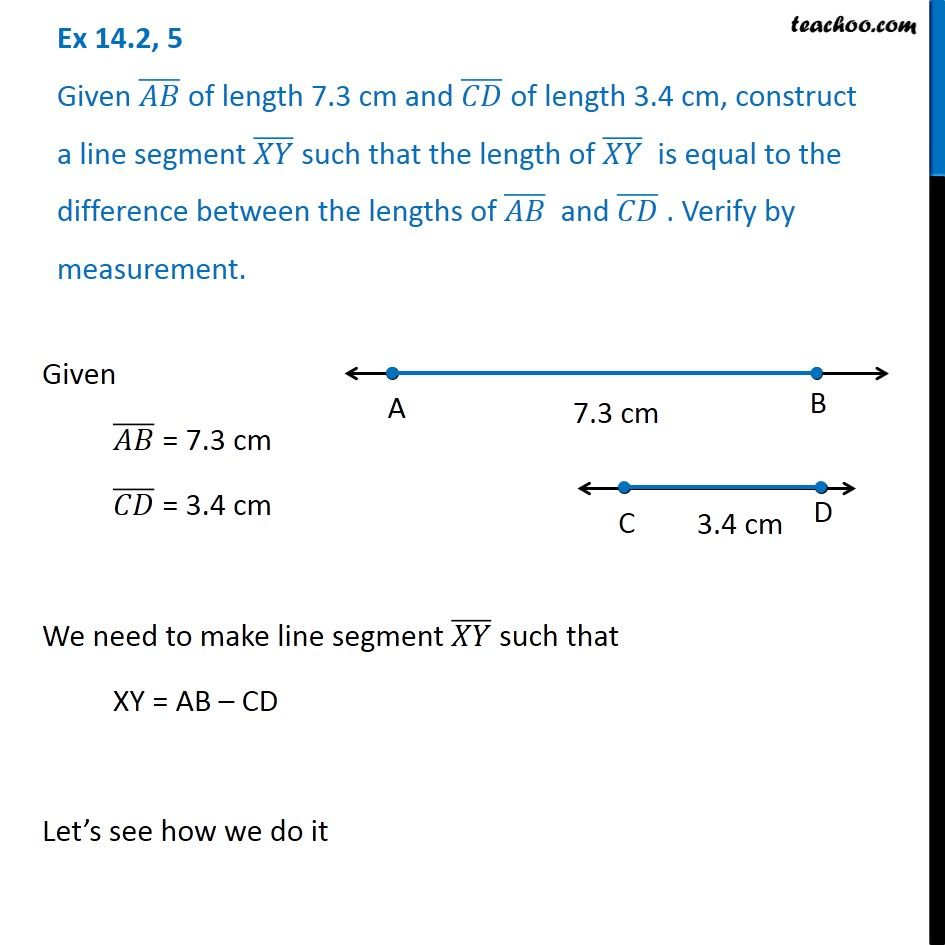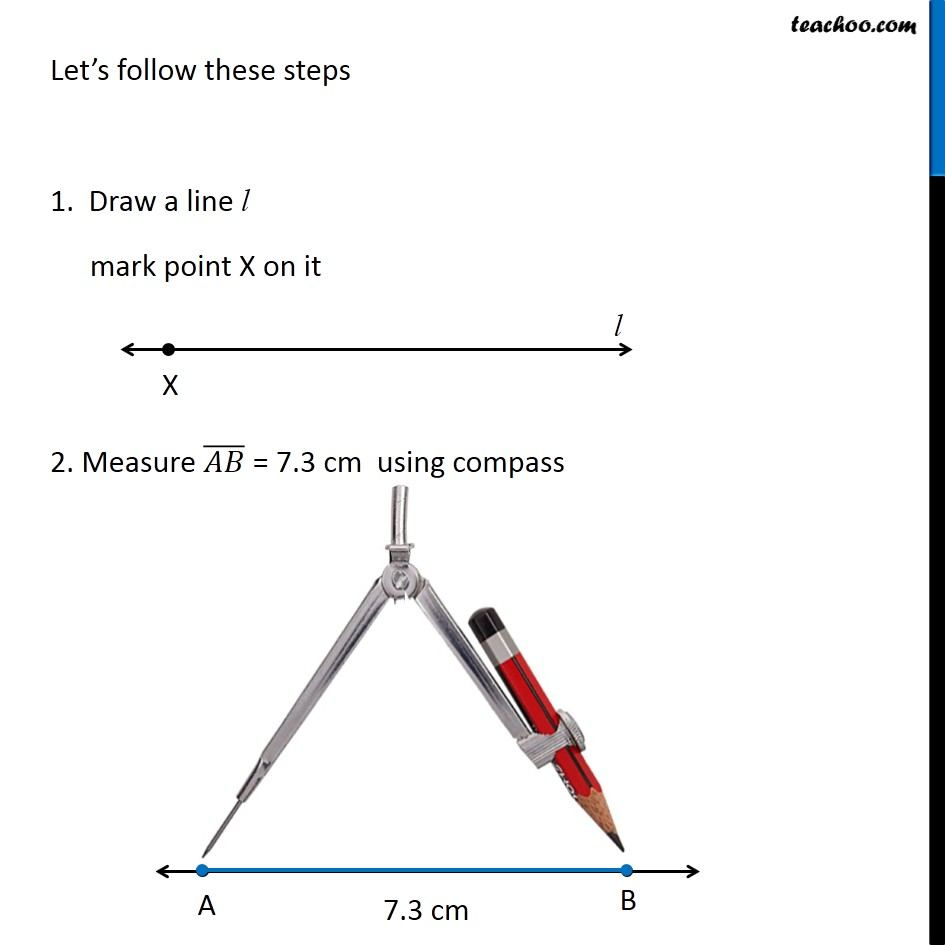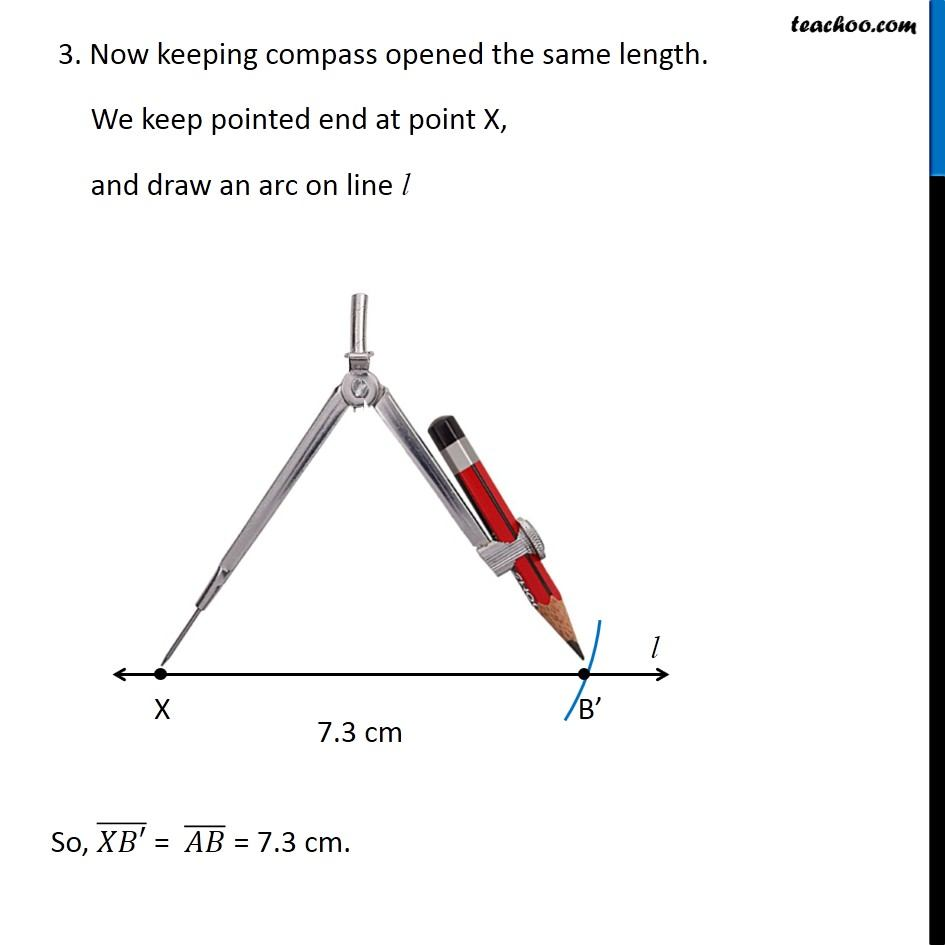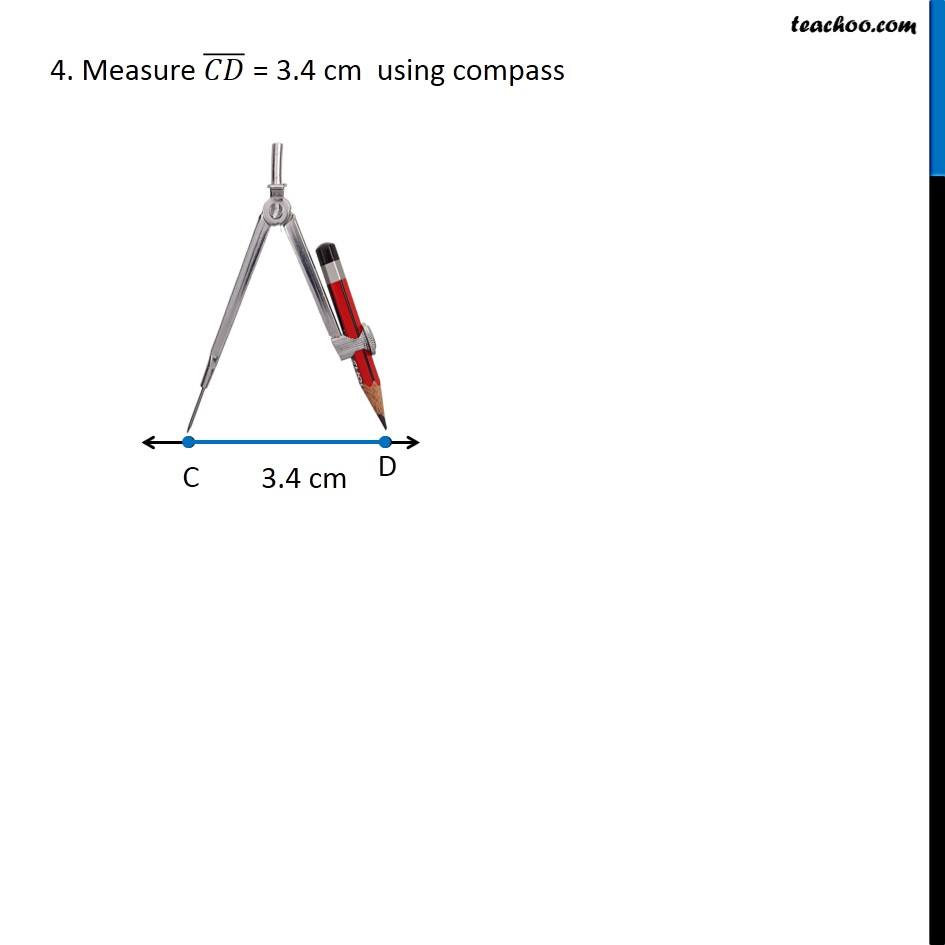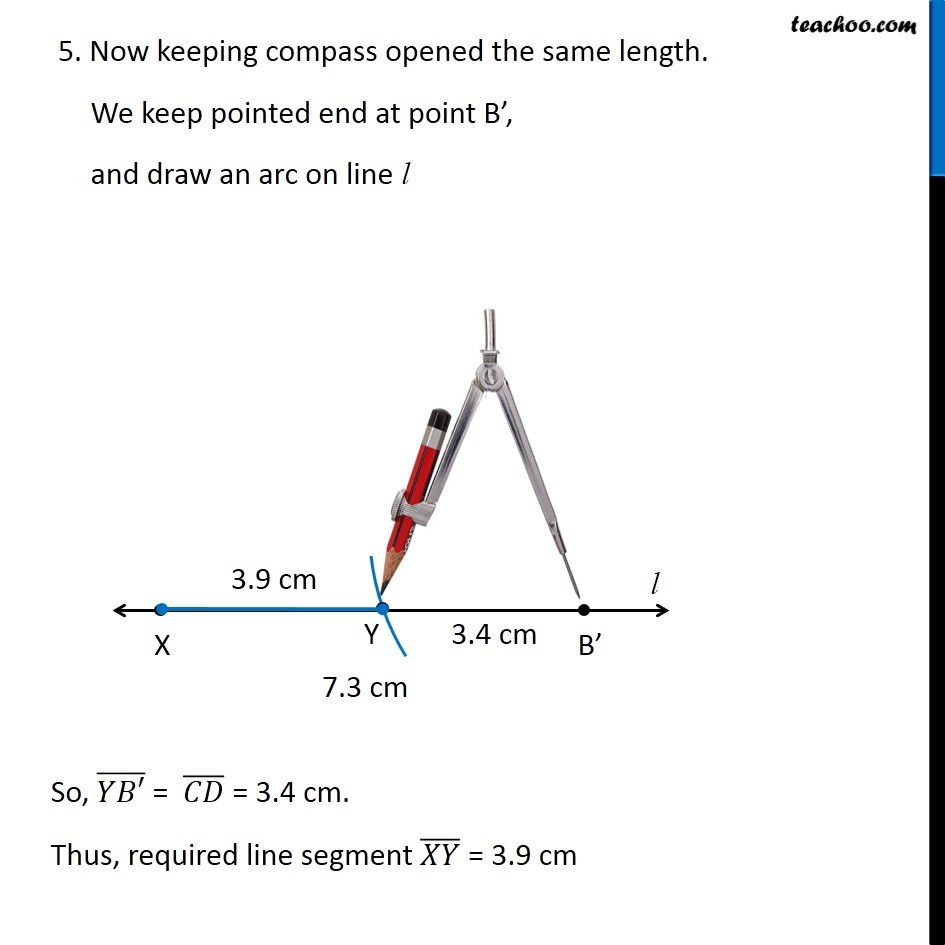Get live Maths 1-on-1 Classs - Class 6 to 12

### Transcript

Ex 14.2, 5 Given (𝐴𝐵) ̅ of length 7.3 cm and (𝐶𝐷) ̅ of length 3.4 cm, construct a line segment (𝑋𝑌) ̅ such that the length of (𝑋𝑌) ̅ is equal to the difference between the lengths of (𝐴𝐵) ̅ and (𝐶𝐷) ̅ . Verify by measurement. Given (𝐴𝐵) ̅ = 7.3 cm (𝐶𝐷) ̅ = 3.4 cm We need to make line segment (𝑋𝑌) ̅ such that XY = AB – CD Let’s see how we do it Let’s follow these steps 1. Draw a line l mark point X on it 2. Measure (𝐴𝐵) ̅ = 7.3 cm using compass 3. Now keeping compass opened the same length. We keep pointed end at point X, and draw an arc on line l So, (𝑋𝐵′) ̅ = (𝐴𝐵) ̅ = 7.3 cm. 4. Measure (𝐶𝐷) ̅ = 3.4 cm using compass 5. Now keeping compass opened the same length. We keep pointed end at point B’, and draw an arc on line l So, (𝑌𝐵′) ̅ = (𝐶𝐷) ̅ = 3.4 cm. Thus, required line segment (𝑋𝑌) ̅ = 3.9 cm